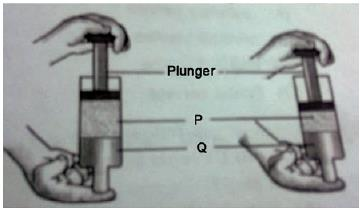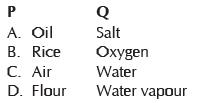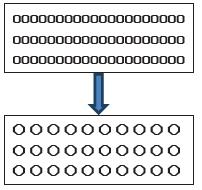Courses

# Olympiad Test: Matter - 2

## 20 Questions MCQ Test Science Olympiad for Class 5 | Olympiad Test: Matter - 2

Description
This mock test of Olympiad Test: Matter - 2 for Class 5 helps you for every Class 5 entrance exam. This contains 20 Multiple Choice Questions for Class 5 Olympiad Test: Matter - 2 (mcq) to study with solutions a complete question bank. The solved questions answers in this Olympiad Test: Matter - 2 quiz give you a good mix of easy questions and tough questions. Class 5 students definitely take this Olympiad Test: Matter - 2 exercise for a better result in the exam. You can find other Olympiad Test: Matter - 2 extra questions, long questions & short questions for Class 5 on EduRev as well by searching above.
QUESTION: 1

Solution:
QUESTION: 2

Solution:
QUESTION: 3

### Husk can be removed from the grain by:

Solution:
QUESTION: 4

Diwali is a famous festival of India. In this festival people burn crackers. When these crackers burn, they turn into ashes. What is such burning of crackers an example of?

Solution:
QUESTION: 5

Matter is made up of these particles:

Solution:
QUESTION: 6

Melting is the opposite of:

Solution:
QUESTION: 7

The difference between boiling and evaporation is:

Solution:
QUESTION: 8

Two substances, P and Q, were used to fill a syringe. The plunger was then pushed in as shown in the diagram. Which of the following would most likely be P and which would be Q?Solution:
QUESTION: 9

Gases consist of particles that:

Solution:
QUESTION: 10

Which change of state occurs when particles in a solid begin to move slowly past each other?Solution:
QUESTION: 11

There is a gold chain weighing 100 g. If the jeweller melts 100 g of pure gold andsells it, he will get:

Solution:
QUESTION: 12

Which of the followings is a physical change?

Solution:
QUESTION: 13

What determines the speed of the atoms and molecules of a particular substance?

Solution:
QUESTION: 14

When ice melts to form water, energy.

Solution:
QUESTION: 15

The ability to change or to move matter is referred to as:

Solution:
QUESTION: 16

As the temperature of a fixed amount of gas at constant volume decreases, itspressure .

Solution:
QUESTION: 17

As the volume of a fixed amount of gas at constant temperature decreases, its pressure .

Solution:
QUESTION: 18

Mist seen often around ice cubes. Which of the following is a probable reason?

Solution:
QUESTION: 19

Which state of matter has particles able to slide past each other, yet still packedtogether?

Solution:
QUESTION: 20

The most common state of matter in the universe is called:

Solution: